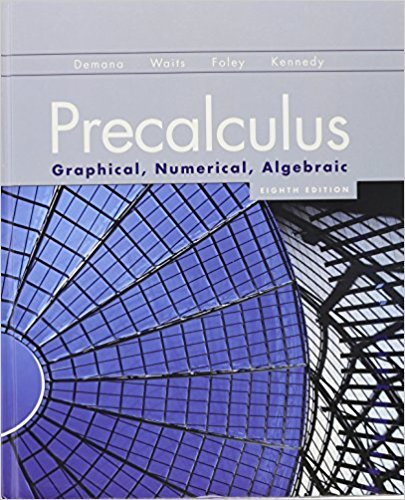×
×

# Solutions for Chapter 1.2: Functions and Graphs## Full solutions for Precalculus: Graphical, Numerical, Algebraic | 8th Edition

ISBN: 9780321656933Solutions for Chapter 1.2: Functions and Graphs

Solutions for Chapter 1.2
4 5 0 330 Reviews
27
2
##### ISBN: 9780321656933

Chapter 1.2: Functions and Graphs includes 97 full step-by-step solutions. Since 97 problems in chapter 1.2: Functions and Graphs have been answered, more than 41865 students have viewed full step-by-step solutions from this chapter. This textbook survival guide was created for the textbook: Precalculus: Graphical, Numerical, Algebraic, edition: 8th Edition. This expansive textbook survival guide covers the following chapters and their solutions. Precalculus: Graphical, Numerical, Algebraic was written by and is associated to the ISBN: 9780321656933.

Key Calculus Terms and definitions covered in this textbook
• Circular functions

Trigonometric functions when applied to real numbers are circular functions

• Compounded annually

See Compounded k times per year.

• Constant

A letter or symbol that stands for a specific number,

• Distance (in a coordinate plane)

The distance d(P, Q) between P(x, y) and Q(x, y) d(P, Q) = 2(x 1 - x 2)2 + (y1 - y2)2

• DMS measure

The measure of an angle in degrees, minutes, and seconds

• Expanded form

The right side of u(v + w) = uv + uw.

• Factor

In algebra, a quantity being multiplied in a product. In statistics, a potential explanatory variable under study in an experiment, .

• Finite series

Sum of a finite number of terms.

• Geometric sequence

A sequence {an}in which an = an-1.r for every positive integer n ? 2. The nonzero number r is called the common ratio.

• Half-life

The amount of time required for half of a radioactive substance to decay.

• Histogram

A graph that visually represents the information in a frequency table using rectangular areas proportional to the frequencies.

• Inverse variation

See Power function.

• Linear regression

A procedure for finding the straight line that is the best fit for the data

• Negative angle

Angle generated by clockwise rotation.

• Nonsingular matrix

A square matrix with nonzero determinant

• Polynomial in x

An expression that can be written in the form an x n + an-1x n-1 + Á + a1x + a0, where n is a nonnegative integer, the coefficients are real numbers, and an ? 0. The degree of the polynomial is n, the leading coefficient is an, the leading term is anxn, and the constant term is a0. (The number 0 is the zero polynomial)

The graph in three dimensions of a seconddegree equation in three variables.

• Real number

Any number that can be written as a decimal.

• Solution of an equation or inequality

A value of the variable (or values of the variables) for which the equation or inequality is true

• Term of a polynomial (function)

An expression of the form anxn in a polynomial (function).

×